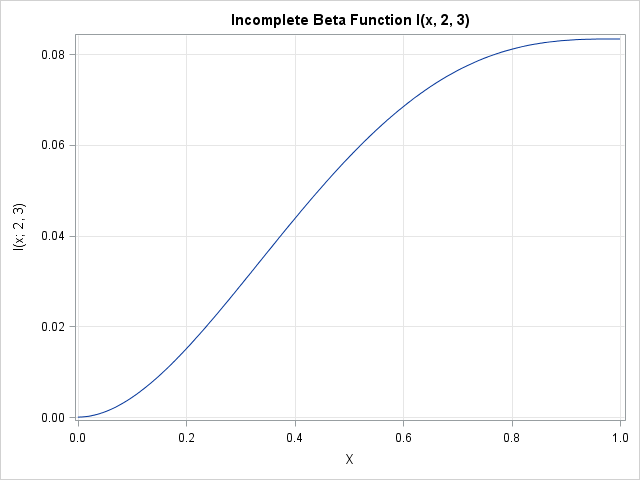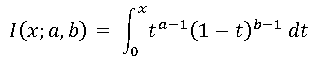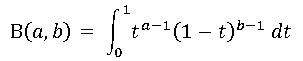While sorting through an old pile of papers, I discovered notes from a 2012 SAS conference that I had attended. Next to the abstract for one presentation, I had scrawled a note to myself that read "BLOG about the incomplete beta function!" Okay, Rick, whatever you say!

In statistics, the incomplete beta function arises during the computation of confidence intervals for order statistics. Order statistics include the minimum value in a sample, the maximum value, the quartiles, the deciles, and so on. From the observed sample, it is possible to use the incomplete beta function to estimate confidence intervals for the quantiles of the population from which the sample was drawn.

At the 2012 conference, I attended a talk in which the presenter lamented that he was not able to find the incomplete beta function in the SAS documentation. Consequently, part of his presentation showed ways to approximate the incomplete beta function and estimate the statistic.

I wrote the note to myself because I realized that there is an easy way to compute the incomplete beta function in SAS. However, this fact is not obvious from the SAS documentation because—as sometimes happens—people in different fields assign different names for the same function.

### Same function, different name

The incomplete beta function is the name given to a special function that arises in numerical analysis and differential equations. It is "incomplete" in the sense that it is defined as the integral from zero to x of some integrand that depends on two-parameters, a and b:In statistics, a normalized version of the function arises more often: the cumulative distribution function (CDF) for the beta distribution. They are the same function, except that the CDF of the beta distribution is normalized so that the integral of the function over [0, 1] is unity. The normalization is accomplished by using the complete beta function, Β(a,b), which is also known as the Euler integral of the first kind:Consequently, the three relevant functions are as follows:

• The complete beta function, Β(a,b), which in SAS software is computed by using the BETA function
• The CDF of the beta distribution, which in SAS software is computed by using the CDF function: CDF("Beta",x,a,b). Notice that the CDF is equal to I(x; a, b) / Β(a,b).
• The incomplete beta function, which is not a built-in function, but can be trivially computed as the product of the previous two functions: I(x,a,b) = Β(a,b)*CDF("Beta",x,a,b)

### A simple example

Suppose that you want to compute and plot the incomplete beta function for the parameters a=2 and b=3. The following SAS/IML statements compute the function:

```proc iml; a = 2; b = 3; x = do(0, 1, 0.01); IBF = Beta(a, b)*CDF("Beta", x, a, b);```

A graph of the function is shown at the beginning of this article. You can see the classic sigmoidal shape that is associated with cumulative probability densities.

SAS is a large, comprehensive, software package that contains many commonly used functions. You might never need the incomplete beta function or its relatives in your work, but there is an important lesson to learn from this exercise. Namely, if you can't find something in the SAS documentation, ask Technical Support or post a question to a relevant SAS Support Community. What you are looking for might be available, but it could be called by a different name.

ShareDistinguished Researcher in Computational Statistics

Rick Wicklin, PhD, is a distinguished researcher in computational statistics at SAS and is a principal developer of SAS/IML software. His areas of expertise include computational statistics, simulation, statistical graphics, and modern methods in statistical data analysis. Rick is author of the books Statistical Programming with SAS/IML Software and Simulating Data with SAS.

1.•